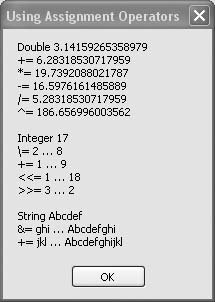# Recipe 6.1. Using Compact Operator Notation

#### Problem

You want to write compact, efficient code using the latest syntax available for assignment operators.

#### Solution

Sample code folder: Chapter 06\CompactOperators

Visual Basic 2005 now lets you use the same compact assignment notation for some math operations that has been used in the C and C# languages for many years.

There are several compact assignment operators, and they all work the same way. The variable to the left of the operator is used both as a source value and as a destination for the results of the operation. The operators are listed in Table 6-1.

Operator

Description

^=

Exponentiation

*=

Multiplication

/=

Division

\=

Integer division

+=

-=

Subtraction

<<=

Shift left

>>=

Shift right

&=

Comparison

#### Discussion

Consider the following program statement, which increments the variable count:

` count = count + 1 `

The variable count is repeated twice in this simple line of code, once to retrieve its value and once to assign the results of adding 1 to the value. The new, more efficient compact assignment syntax uses the variable's name just once:

` count += 1 `

The compact assignment operator += causes the variable to be used both as the source of the value to be operated on and as the destination for the result.

The following sample code demonstrates all of the operators listed in Table 6-1:

` Dim result As New System.Text.StringBuilder Dim testDouble As Double = Math.PI result.Append("Double ").AppendLine(testDouble) testDouble += Math.PI result.Append("+= ").AppendLine(testDouble) testDouble *= Math.PI result.Append("*= ").AppendLine(testDouble) testDouble -= Math.PI result.Append("-= ").AppendLine(testDouble) testDouble /= Math.PI result.Append("/= ").AppendLine(testDouble) testDouble ^= Math.PI result.Append("^= ").AppendLine(testDouble) result.AppendLine() Dim testInteger As Integer = 17 result.Append("Integer ").AppendLine(testInteger) testInteger \= 2 result.Append("\= 2 … ").AppendLine(testInteger) testInteger += 1 result.Append("+= 1 … ").AppendLine(testInteger) testInteger <<= 1 result.Append("<<= 1 … ").AppendLine(testInteger) testInteger >>= 3 result.Append(">>= 3 … ").AppendLine(testInteger) result.AppendLine() Dim testString As String = "Abcdef" result.Append("String ").AppendLine(testString) testString &= "ghi" result.Append("&= ghi … ").AppendLine(testString) testString += "jkl" result.Append("+= jkl … ").AppendLine(testString) MsgBox(result.ToString()) `

Figure 6-1 shows the results displayed by this block of code. While many of the operators work on double-precision variables, some work only on integers of various sizes, and the concatenation operator works only on strings.

##### Figure 6-1. The compact assignment operators in actionAlthough the += (addition) operator is overloaded to operate on either numerical variables or strings, your code will be clearer if you use the addition operator only for mathematical operations. For string concatenation, use the &= operator instead. This rule can also help you avoid hidden errors when working with numbers formatted as strings. For instance, consider the following code, which updates an Integer value with numbers stored in strings:

` Dim numberFromStrings As Integer numberFromStrings = "4" numberFromStrings += "3" MsgBox(numberFromStrings) `

When you run this code, it displays "7" in the message box. This works because Visual Basic is "helping you out," automatically converting the strings to Integer values before performing the assignment or addition. If you replace the += operator in that code with the &= operator, the code behaves differently:

` Dim numberFromStrings As Integer numberFromStrings = "4" numberFromStrings &= "3" MsgBox(numberFromStrings) `

This time, the message box displays "43," the concatenation of the two strings. Some of the documentation for the += and &= operators claims that the two are functionally equivalent when working with strings, but this example shows that care should be exercised when using them in mixed string/number situations.Visual Basic 2005 Cookbook: Solutions for VB 2005 Programmers (Cookbooks (OReilly))
ISBN: 0596101775
EAN: 2147483647
Year: 2006
Pages: 400

Similar book on Amazon